# The roof of a single-story storage building, shown in Fig. P2.1, is subjected to a uniformly distributed load of 0.96 kPa over its surface area. Determine the loads acting on the floor beam BE and the girder AC of the framing system. FIG. P2.1, P2.2### Structural Analysis

6th Edition
KASSIMALI + 1 other
Publisher: Cengage,
ISBN: 9781337630931### Structural Analysis

6th Edition
KASSIMALI + 1 other
Publisher: Cengage,
ISBN: 9781337630931

#### Solutions

Chapter 2, Problem 1P
Textbook Problem

## The roof of a single-story storage building, shown in Fig. P2.1, is subjected to a uniformly distributed load of 0.96 kPa over its surface area. Determine the loads acting on the floor beam BE and the girder AC of the framing system.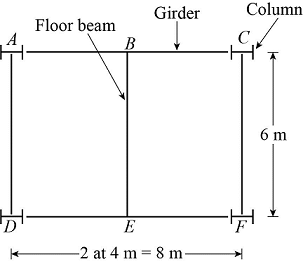FIG. P2.1, P2.2

Expert Solution
To determine

Calculate the loads that is acting on the floor beam BE and girder AC.

The uniformly distributed load acting on the floor beam BE is 3.84kN/m_.

The load acting at A, B, and C on the girder AC are PA=5.76kN_, PB=11.52kN_, and PC=5.76kN_.

### Explanation of Solution

Given information:

The building is a single-story building.

The building is subjected to uniformly distributed load of wUDL=0.96kPa.

Calculation:

Show the roof of the single-story storage building as shown in Figure 1.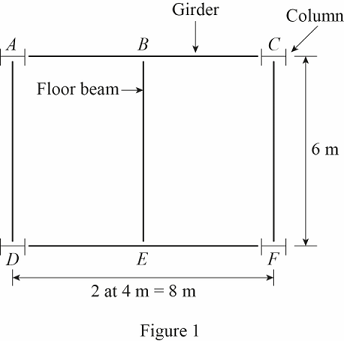Refer Figure 1.

The columns are denoted by A, C, D, and F.

The floor beam is denoted by BE.

The girders are denoted by AC and DF.

Show the tributary area of the floor beam BE as shown in Figure 2.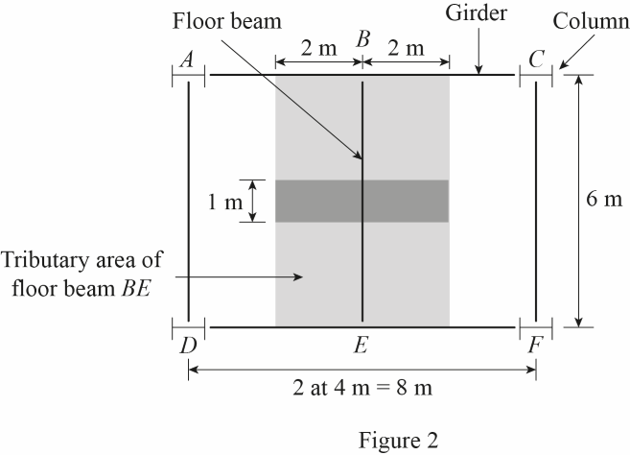Refer Figure 2.

The tributary area of the floor beam BE is denoted by the shaded area.

Calculate the tributary area of the floor beam BE (A1) as follows:

A1=4m×6m=24m2

The length of the floor beam BE is L=6m.

Calculate the uniformly distributed load (W1) acting on the floor beam BE as follows:

W1=wUDL×A1L

Substitute 0.96kPa for wUDL, 6m for L and 24m2 for A1.

W1=0.96kPa×24m26m=3.84kN/m

Show the uniformly distributed load acting on the floor beam BE as shown in Figure 3.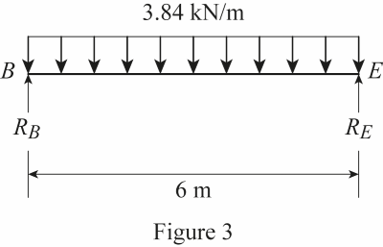Refer Figure 3.

The reactions at B and E are denoted by RB and RE respectively.

Calculate the value of RB and RE using the relation:

RB=RE=3.84kN/m×6m2RB=RE=11.52kN

Show the uniformly distributed load acting on the floor beam BE as shown in Figure 4.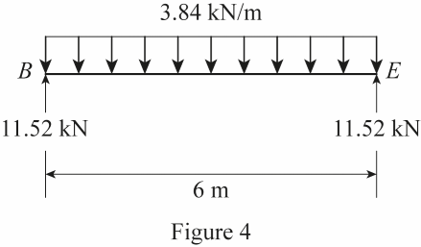Refer Figure 4.

Thus, the uniformly distributed load acting on the floor beam BE is 3.84kN/m_.

Show the tributary area of the girder AC as shown in Figure 5.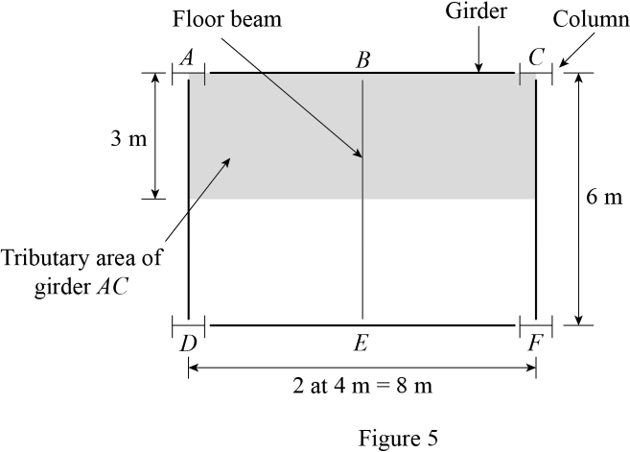Refer Figure 5.

The tributary area of the girder AC is denoted by the shaded area.

Calculate the tributary area of the girder AC (A2) as follows:

A2=8m×3m=24m2

Calculate the load (W2) acting on the girder AC as follows:

W2=wUDL×A2

Substitute 0.96kPa for wUDL and 24m2 for A2.

W2=0.96kPa×24m2=23.04kN

The total load acting on the tributary area of the girder AC is 23.04kN.

Almost half the load acts at the junction of the floor beam BE and the girder AC. Then,

The load acting at B is 11.52kN.

The remaining half of the load acts equally on the column A and C. Then,

The load acting at A is 5.76kN and the load acting at C is 5.76kN.

Show the load acting on the girder AC as shown in Figure 6.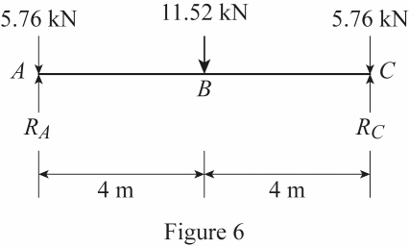Refer Figure 6.

The reactions at A and C are denoted by RA and RC respectively.

Calculate the value of RA and RC using the relation:

RA=RC=(5.76+11.52+5.762)kNRA=RC=11.52kN

Show the load acting on the girder AC as shown in Figure 7.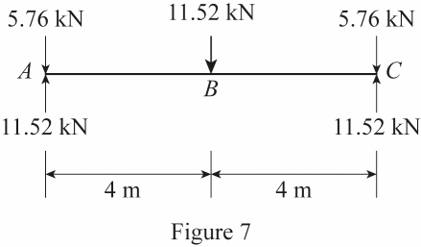Refer Figure 7.

Thus, the load acting at A, B, and C on the girder AC are PA=5.76kN_, PB=11.52kN_, and PC=5.76kN_.

### Want to see more full solutions like this?

Subscribe now to access step-by-step solutions to millions of textbook problems written by subject matter experts!

### Want to see more full solutions like this?

Subscribe now to access step-by-step solutions to millions of textbook problems written by subject matter experts!

Find more solutions based on key concepts
Compute the interest earned on the deposits made in Problem 20.1.

Engineering Fundamentals: An Introduction to Engineering (MindTap Course List)

List technologies that processor chips often include.

Enhanced Discovering Computers 2017 (Shelly Cashman Series) (MindTap Course List)

What does SPC stand for?

Precision Machining Technology (MindTap Course List)

What is the purpose of the NEC?

EBK ELECTRICAL WIRING RESIDENTIAL

What is Port Address Translation (PAT) and how does it work?

Principles of Information Security (MindTap Course List)

Create the basic ERD for the database shown in Figure Q3.8.

Database Systems: Design, Implementation, & Management

If your motherboard supports ECC DDR3 memory, can you substitute non-ECC DDR3 memory?

A+ Guide to Hardware (Standalone Book) (MindTap Course List)

Describe the three classifications of burns.

Welding: Principles and Applications (MindTap Course List)